## Wednesday, June 24, 2020

### Simultaneous estimation of the transition rate and Pagel's λ under the Mk model

Recently, a colleague contacted me to ask me about his analysis fitting the Mk model with a tree transformation - such as the λ transform of Pagel (1999). This can be done pretty easily using geiger's `fitDiscrete` function.

For some reason, I've always looked at this endeavor with some degree of suspicion. This is because λ, by some definitions, is a metric of phylogenetic signal - and thus it seemed logical to assume that λ might be confounded with the transition rate between states, q.

In the limiting case in which q is very high relative to the tree length it would seem that this would have to be true because at that point the data become random with respect to the topology and thus would have the same probability under a low value of λ and a relatively low q, as with high values of both parameters.

Nonetheless, I was curious enough to investigate whether or not it is possible to simultaneously estimate q & λ - and under what conditions.

The first think I did (after loading my packages) was simulate a tree & discrete character under a modest transition rate between states:

``````library(phytools)
library(geiger)
tree<-pbtree(n=100,scale=1)
Q<-matrix(c(-1,1,1,-1),2,2,dimnames=list(0:1,0:1))
x<-sim.Mk(tree,Q)
``````

Here's my simulated data:

``````plotTree(tree,ftype="off",type="fan",part=0.5,
mar=c(3.1,1.1,1.1,1.1),lwd=1)
axis(1,at=c(0,0.5,1))
cols<-setNames(palette()[2:3],levels(x))
tiplabels(pie=to.matrix(x,levels(x)),piecol=cols,
cex=0.3)
``````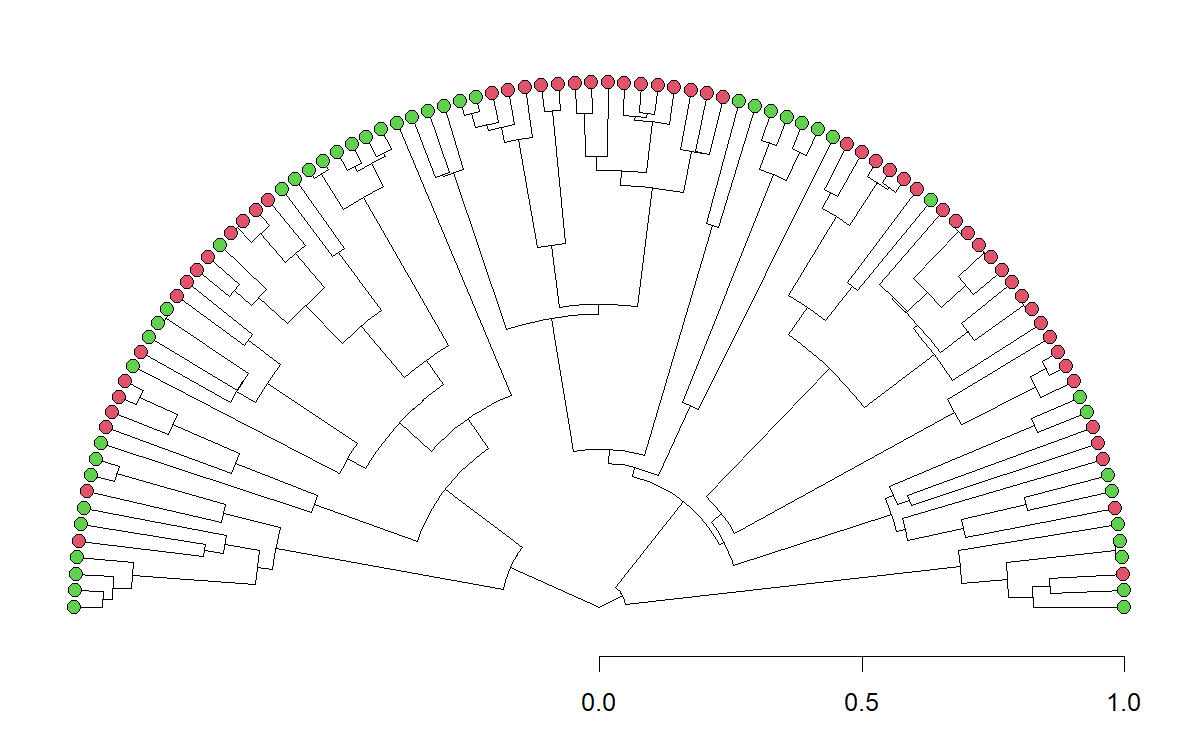Next, let's fit a λ model

``````fit.er<-fitDiscrete(tree,x,model="ER")
fit.lambda<-fitDiscrete(tree,x,model="ER",
transform="lambda")
``````
``````fit.er
``````
``````## GEIGER-fitted comparative model of discrete data
##  fitted Q matrix:
##               0         1
##     0 -1.118624  1.118624
##     1  1.118624 -1.118624
##
##  model summary:
##  log-likelihood = -45.181453
##  AIC = 92.362907
##  AICc = 92.403723
##  free parameters = 1
##
## Convergence diagnostics:
##  optimization iterations = 100
##  failed iterations = 0
##  number of iterations with same best fit = 100
##  frequency of best fit = 1.00
##
##  object summary:
##  'lik' -- likelihood function
##  'bnd' -- bounds for likelihood search
##  'res' -- optimization iteration summary
##  'opt' -- maximum likelihood parameter estimates
``````
``````fit.lambda
``````
``````## GEIGER-fitted comparative model of discrete data
##  fitted Q matrix:
##               0         1
##     0 -1.118624  1.118624
##     1  1.118624 -1.118624
##
##  fitted 'lambda' model parameter:
##  lambda = 1.000000
##
##  model summary:
##  log-likelihood = -45.181453
##  AIC = 94.362907
##  AICc = 94.486618
##  free parameters = 2
##
## Convergence diagnostics:
##  optimization iterations = 100
##  failed iterations = 41
##  number of iterations with same best fit = NA
##  frequency of best fit = NA
##
##  object summary:
##  'lik' -- likelihood function
##  'bnd' -- bounds for likelihood search
##  'res' -- optimization iteration summary
##  'opt' -- maximum likelihood parameter estimates
``````

At least in this case, the estimated value of λ is identical to its simulated value, and thus does not affect the fit or model parameter estimates for our Mk model.

Nonetheless, let's plot our likelihood surface to see how if they are correlated as I had suspected:

``````m<-60
q<-seq(0.1*fit.lambda\$opt\$q12,1.5*fit.lambda\$opt\$q12,
length.out=m)
lambda<-seq(0,phytools:::maxLambda(tree),
length.out=m)
logL<-matrix(NA,m,m)
for(i in 1:m) for(j in 1:m)
logL[i,j]<-logLik(fitMk(
phytools:::lambdaTree(tree,lambda[i]),
x,fixedQ=Q*q[j],pi="fitzjohn"))
contour(lambda,q,logL,nlevels=50,
xlab=expression(lambda),ylab="q",bty="l",
font.lab=3)
title(main=expression(paste("Likelihood surface for q and ",
lambda)))
points(fit.lambda\$opt\$lambda,fit.lambda\$opt\$q12,cex=1.5,
pch=19,col="blue")
ml.q<-sapply(lambda,function(lambda,tree,x)
fitMk(phytools:::lambdaTree(tree,lambda),
x,model="ER",pi="fitzjohn")\$rates,tree=tree,
x=x)
lines(lambda,ml.q,col=make.transparent("blue",0.5),
lwd=3)
legend("topleft",c(expression(paste("ML(q,",lambda,")")),
expression(paste("ML(q|",lambda,")"))),
lwd=c(NA,3),pch=c(19,NA),
col=c("blue","blue"),
bg="white",pt.cex=1.5,pt.lwd=2)
``````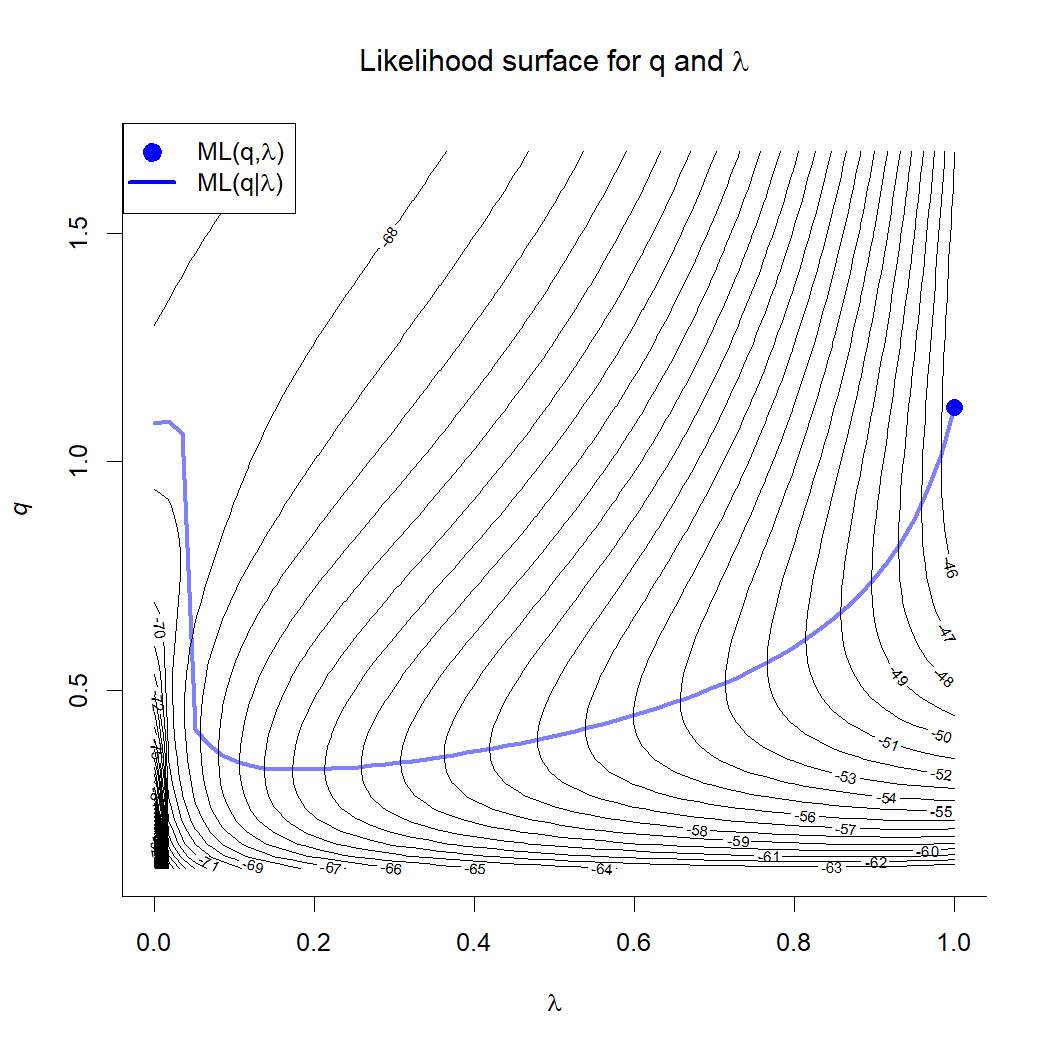The curved line gives the conditional ML value of q for each value of λ represented on the surface.

Interestingly, q and λ seem to be somewhat correlated - but perhaps not as much as I'd expected a priori

Now, for fun, let's try again but with a 10 × value of q - the transition rate between states.

``````y<-sim.Mk(tree,10*Q)
plotTree(tree,ftype="off",type="fan",part=0.5,
mar=c(3.1,1.1,1.1,1.1),lwd=1)
axis(1,at=c(0,0.5,1))
cols<-setNames(palette()[5:6],levels(y))
tiplabels(pie=to.matrix(y,levels(y)),piecol=cols,
cex=0.3)
``````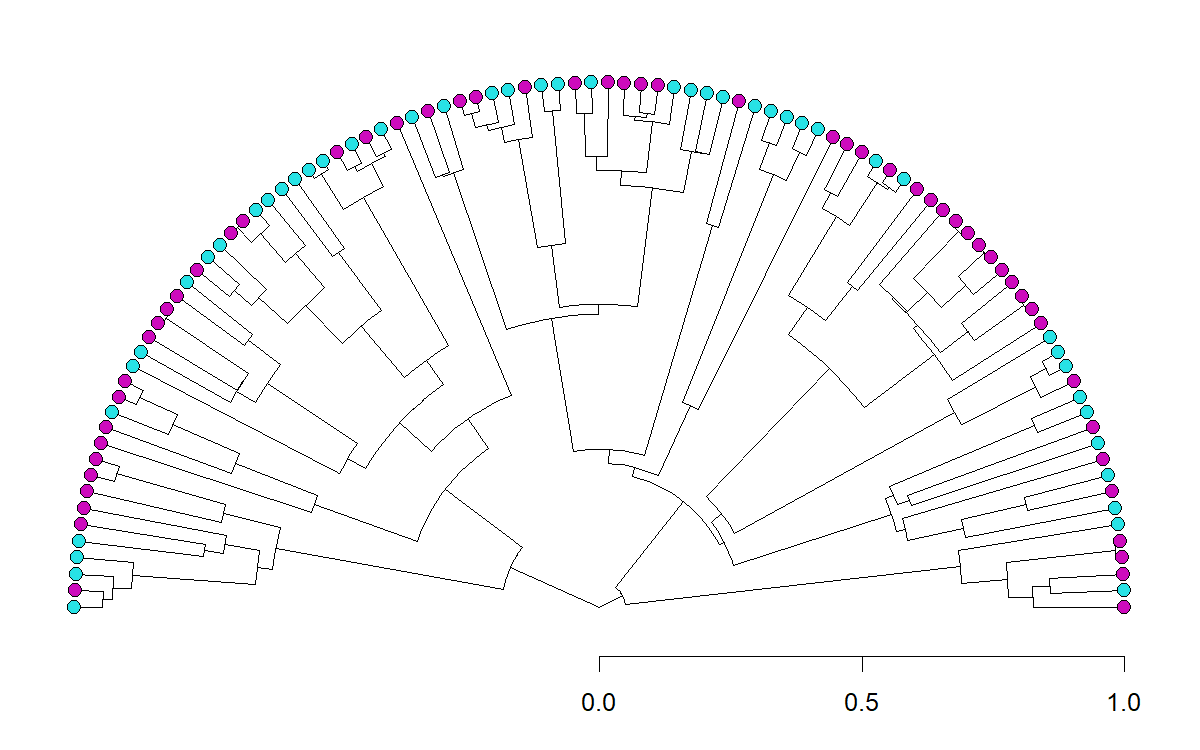``````fit.er<-fitDiscrete(tree,y,model="ER")
fit.lambda<-fitDiscrete(tree,y,model="ER",
transform="lambda")
``````
``````fit.er
``````
``````## GEIGER-fitted comparative model of discrete data
##  fitted Q matrix:
##               0         1
##     0 -9.308253  9.308253
##     1  9.308253 -9.308253
##
##  model summary:
##  log-likelihood = -65.758662
##  AIC = 133.517325
##  AICc = 133.558141
##  free parameters = 1
##
## Convergence diagnostics:
##  optimization iterations = 100
##  failed iterations = 0
##  number of iterations with same best fit = 100
##  frequency of best fit = 1.00
##
##  object summary:
##  'lik' -- likelihood function
##  'bnd' -- bounds for likelihood search
##  'res' -- optimization iteration summary
##  'opt' -- maximum likelihood parameter estimates
``````
``````fit.lambda
``````
``````## GEIGER-fitted comparative model of discrete data
##  fitted Q matrix:
##               0         1
##     0 -9.308253  9.308253
##     1  9.308253 -9.308253
##
##  fitted 'lambda' model parameter:
##  lambda = 1.000000
##
##  model summary:
##  log-likelihood = -65.758662
##  AIC = 135.517325
##  AICc = 135.641036
##  free parameters = 2
##
## Convergence diagnostics:
##  optimization iterations = 100
##  failed iterations = 41
##  number of iterations with same best fit = NA
##  frequency of best fit = NA
##
##  object summary:
##  'lik' -- likelihood function
##  'bnd' -- bounds for likelihood search
##  'res' -- optimization iteration summary
##  'opt' -- maximum likelihood parameter estimates
``````

Even though we increased the transition rate, q, quite a lot, our ML value of λ remains totally unchanged.

Let's visualize the likelihood surface again. Having looked ahead (that is, tried this in advance of building the post), I know that our likelihood surface is going to look a bit weird, so I will only estimate it for 0.6 < λ < 1.

``````m<-60
q<-seq(0.1*fit.lambda\$opt\$q12,1.5*fit.lambda\$opt\$q12,
length.out=m)
lambda<-seq(0.6,phytools:::maxLambda(tree),
length.out=m)
logL<-matrix(NA,m,m)
for(i in 1:m) for(j in 1:m)
logL[i,j]<-logLik(fitMk(
phytools:::lambdaTree(tree,lambda[i]),
y,fixedQ=Q*q[j],pi="fitzjohn"))
contour(lambda,q,logL,nlevels=50,
xlab=expression(lambda),ylab="q",bty="l",
font.lab=3)
title(main=expression(paste("Likelihood surface for q and ",
lambda)))
points(fit.lambda\$opt\$lambda,fit.lambda\$opt\$q12,cex=1.5,
pch=19,col="blue")
ml.q<-sapply(lambda,function(lambda,tree,x)
fitMk(phytools:::lambdaTree(tree,lambda),
x,model="ER",pi="fitzjohn")\$rates,tree=tree,
x=y)
lines(lambda,ml.q,col=make.transparent("blue",0.5),
lwd=3)
legend("topleft",c(expression(paste("ML(q,",lambda,")")),
expression(paste("ML(q|",lambda,")"))),
lwd=c(NA,3),pch=c(19,NA),
col=c("blue","blue"),
bg="white",pt.cex=1.5,pt.lwd=2)
``````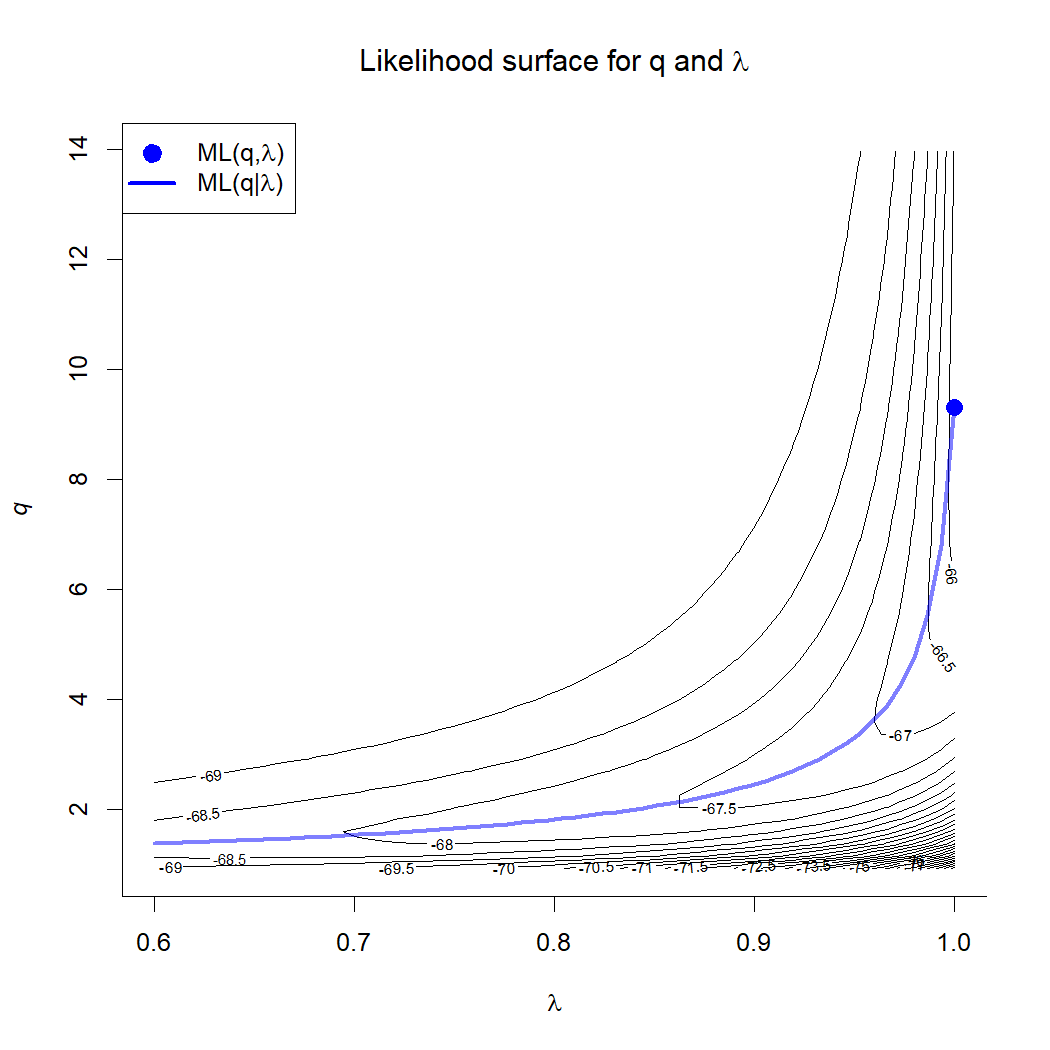Here, as before, we see a prominent ridge on the likelihood surface - but one that nonetheless leads up to λ = 1.

Lastly, let's simulate an intermediate value of λ - let's say, λ = 0.6 - and then see if it can be estimated from a simulated dataset for a discrete trait.

``````z<-sim.Mk(phytools:::lambdaTree(tree,0.6),Q)
plotTree(tree,ftype="off",type="fan",part=0.5,
mar=c(3.1,1.1,1.1,1.1),lwd=1)
axis(1,at=c(0,0.5,1))
cols<-setNames(palette()[5:6],levels(y))
tiplabels(pie=to.matrix(z,levels(z)),piecol=cols,
cex=0.3)
``````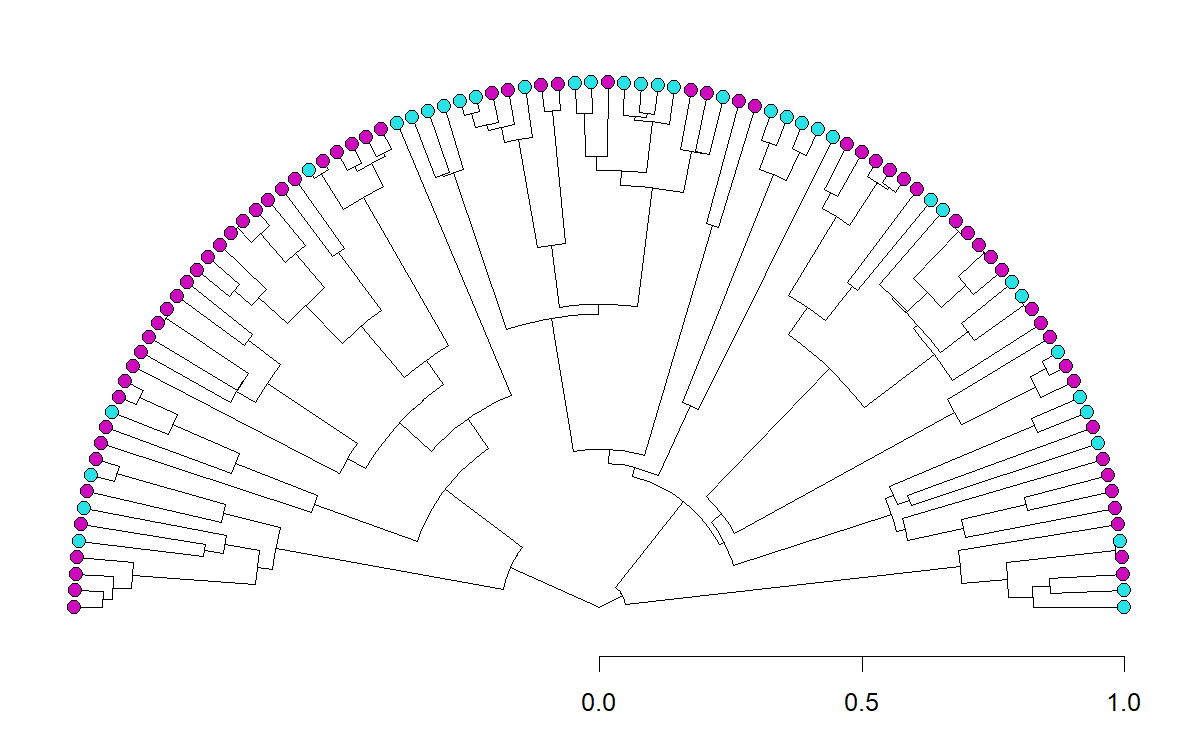``````fit.er<-fitDiscrete(tree,z,model="ER")
fit.lambda<-fitDiscrete(tree,z,model="ER",
transform="lambda")
``````
``````fit.er
``````
``````## GEIGER-fitted comparative model of discrete data
##  fitted Q matrix:
##               0         1
##     0 -2.552545  2.552545
##     1  2.552545 -2.552545
##
##  model summary:
##  log-likelihood = -64.272856
##  AIC = 130.545712
##  AICc = 130.586528
##  free parameters = 1
##
## Convergence diagnostics:
##  optimization iterations = 100
##  failed iterations = 0
##  number of iterations with same best fit = 100
##  frequency of best fit = 1.00
##
##  object summary:
##  'lik' -- likelihood function
##  'bnd' -- bounds for likelihood search
##  'res' -- optimization iteration summary
##  'opt' -- maximum likelihood parameter estimates
``````
``````fit.lambda
``````
``````## GEIGER-fitted comparative model of discrete data
##  fitted Q matrix:
##                0          1
##     0 -0.8316731  0.8316731
##     1  0.8316731 -0.8316731
##
##  fitted 'lambda' model parameter:
##  lambda = 0.765701
##
##  model summary:
##  log-likelihood = -59.455357
##  AIC = 122.910714
##  AICc = 123.034426
##  free parameters = 2
##
## Convergence diagnostics:
##  optimization iterations = 100
##  failed iterations = 42
##  number of iterations with same best fit = NA
##  frequency of best fit = NA
##
##  object summary:
##  'lik' -- likelihood function
##  'bnd' -- bounds for likelihood search
##  'res' -- optimization iteration summary
##  'opt' -- maximum likelihood parameter estimates
``````
``````m<-60
q<-seq(0.1*fit.lambda\$opt\$q12,1.5*fit.lambda\$opt\$q12,
length.out=m)
lambda<-seq(0,phytools:::maxLambda(tree),
length.out=m)
logL<-matrix(NA,m,m)
for(i in 1:m) for(j in 1:m)
logL[i,j]<-logLik(fitMk(
phytools:::lambdaTree(tree,lambda[i]),
z,fixedQ=Q*q[j],pi="fitzjohn"))
contour(lambda,q,logL,nlevels=50,
xlab=expression(lambda),ylab="q",bty="l",
font.lab=3)
title(main=expression(paste("Likelihood surface for q and ",
lambda)))
points(fit.lambda\$opt\$lambda,fit.lambda\$opt\$q12,cex=1.5,
pch=19,col="blue")
ml.q<-sapply(lambda,function(lambda,tree,x)
fitMk(phytools:::lambdaTree(tree,lambda),
x,model="ER",pi="fitzjohn")\$rates,tree=tree,
x=z)
lines(lambda,ml.q,col=make.transparent("blue",0.5),
lwd=3)
legend("topleft",c(expression(paste("ML(q,",lambda,")")),
expression(paste("ML(q|",lambda,")"))),
lwd=c(NA,3),pch=c(19,NA),
col=c("blue","blue"),
bg="white",pt.cex=1.5,pt.lwd=2)
``````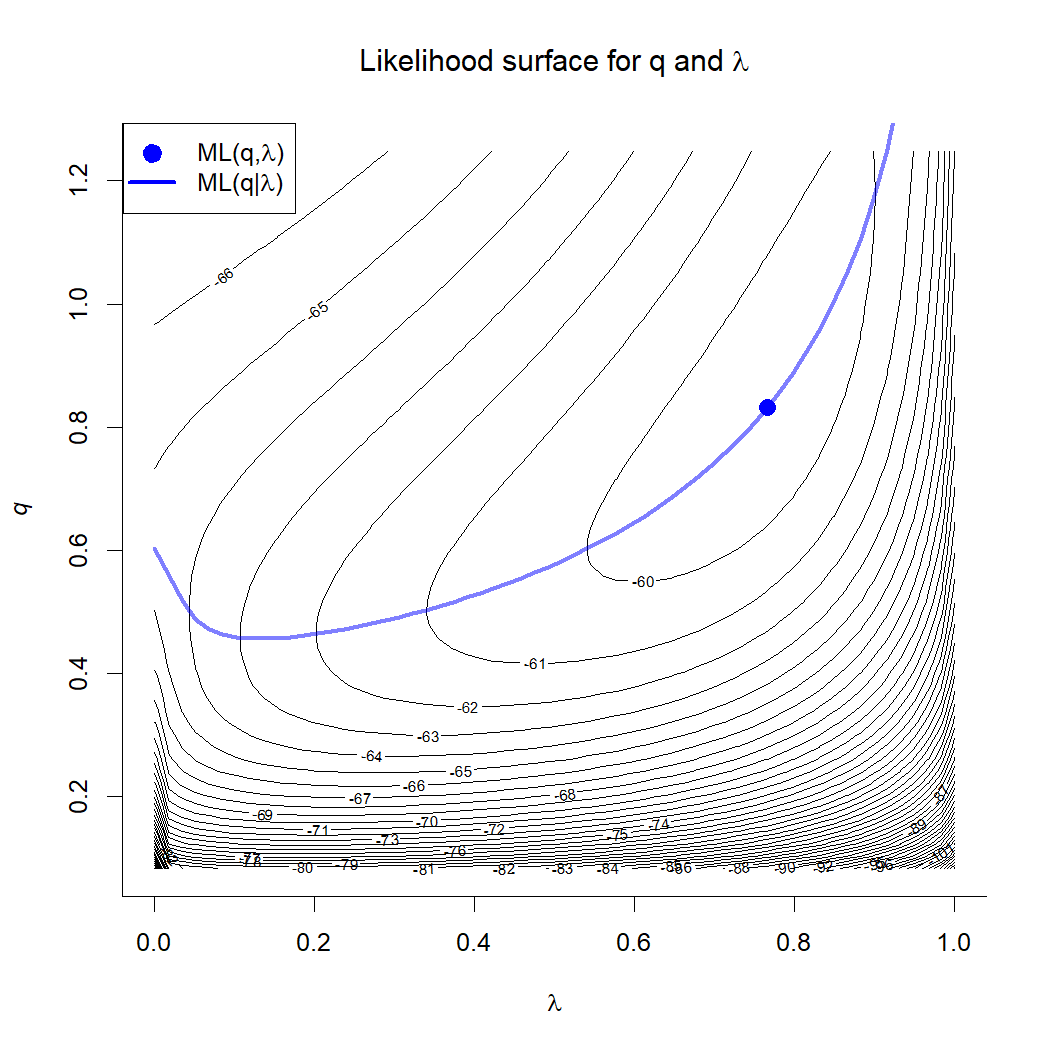I'm not sure how far we can push q & have this continue to hold up - but here for even quite large transition rates between states, it would seem that (contrary to my prediction) q and λ are not totally confounded, and probably can be separately estimated from many datasets.

The interpretation of λ…. That's another story!

#### 1 comment:

1.Dear Liam,

I am trying to plot likelihood surface for fitDiscrete() to see if best model can be easily identified, you seem to say that it might be done easily but I am not able to figure it out.
I have been running several times a model with many (66) parameters, delta transformed, 100<niter<1000 (soon more...).

I was also wondering how to save fit.discrete result to not have to reload the models if especially if we run several big ones?.

Hope it can be useful for others too.

Thanks,

Best wishes

Note: due to the very large amount of spam, all comments are now automatically submitted for moderation.